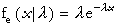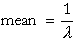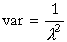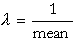Exponential Distribution

#Exponential Distribution

The Exponential Distribution, a special case of the Gamma Distribution, is generally used to model the distribution of times between the occurrence of successive events, such as waiting times or lifetimes. Some examples are: Customers arriving at a service facility, calls coming in to a switchboard, and lifetime of an electronic component. In failure analysis, it is used to represent the time between failures assuming a constant failure rate per unit time.

A key property of the Exponential Distribution is that a truncated Exponential Distribution is also exponential. For example, let's suppose we are using it to model the time to failure for a light bulb. After the light bulb has survived for some time t, the future conditional time to failure is still the original exponential distribution.

The Exponential Distribution is defined for all positive values of x and declines exponentially. Its only parameter is lamda, which must be positive. The distribution is:where x>0 and l>0.

The equations for mean and variance are:The equation for the parameter is:The mean must be positive.

Versions: DPL Professional, DPL Enterprise, DPL Portfolio